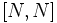# Congruence condition on number of elementary abelian subgroups of prime-cube and prime-fourth order for odd prime

Jump to: navigation, search
This article defines a replacement theorem
View a complete list of replacement theorems| View a complete list of failures of replacement
This article is about a congruence condition.
View other congruence conditions

## Statement

### In terms of a universal congruence condition

Suppose$p$ is an odd prime and$k = 3$ or$k = 4$. Then, the singleton set comprising the elementary abelian group of order$p^k$ is a Collection of groups satisfying a universal congruence condition (?) for the prime$p$.

### Hands-on statement

Let$p$ be an odd prime and$k = 3$ or$k = 4$. Then, if$P$ is a finite$p$-group, the number of elementary abelian subgroups of$P$ of order$p^k$ is either equal to zero or congruent to$1$ modulo$p$.

## Facts used

1. Jonah-Konvisser lemma for elementary abelian-to-normal replacement for prime-cube and prime-fourth order: This states that if$E_1, E_2$ are two distinct elementary abelian normal subgroups of$P$, and$N = E_1E_2$ satisfies$|N'| \le p^2$ or$|N/Z(N)| \le p^2$, then any maximal subgroup of$N$ containing$E_1 \cap E_2$ contains an elementary abelian subgroup of order$p^k$.
2. Support of good lines corollary to line lemma, Jonah-Konvisser line lemma

## Proof

### Preliminary lemma

Given:$p$ odd,$k = 3$ or$k = 4$, and$E_1,E_2$ are normal subgroups of a finite$p$-group both of order$p^k$.$N = E_1E_2$.

To prove:$[N,N]$ has order at most$p^2$ or$N/Z(N)$ has order at most$p^2$.

Proof: Since$N = E_1E_2$ and both$E_1$ and$E_2$ are abelian and normal,$[N,N] = [E_1,E_2] \le E_1 \cap E_2 \le Z(N)$. We consider the cases:

1.$E_1 \cap E_2$ has order at most$p^2$: In this case,$[N,N]$ has order at most$p^2$.
2.$E_1 \cap E_2$ has order$p^3$: In this case,$N = E_1E_2$ has order$p^5$, so$N/Z(N)$ has order$p^2$.

### Main proof

We prove the statement by induction on the order of the finite$p$-group. Here$k = 3$ or$k = 4$.

Given: A finite$p$-group$P$ containing an elementary abelian subgroup of order$p^k$.

To prove: The number of elementary abelian subgroups of$P$ of order$p^k$ is congruent to$1$ modulo$p$.

Proof: If$P$ itself has order$p^k$, the number of such subgroups is$1$. Thus, we assume that the order of$P$ is greater than$p^k$.

1. There is a maximal subgroup$M$ of$P$ containing the elementary abelian subgroup: This follows because the elementary abelian subgroup is proper.
2. There is an elementary abelian subgroup$E$ of$G$ of order$p^k$ (in fact,$E \le M$): This follows from the various equivalent formulations of universal congruence condition for$M$. Explicitly, the number of elementary abelian subgroups of order$p^k$ in$M$ is congruent to$1$ modulo$p$. The action of$G$ on these subgroups by conjugation has orbits of size$1$ or multiples of$p$, so there is at least one orbit of size$1$, and this gives a normal subgroup.
3. Any pair of distinct elementary abelian normal subgroups supports good lines for the collection of elementary abelian subgroups of order$p^k$. In other words, if$E_1,E_2$ are distinct elementary abelian normal subgroups of order$p^k$, any maximal subgroup containing$E_1 \cap E_2$ contains an elementary abelian subgroup of order$p^k$:
1. If$N = E_1E_2$, then either$|N'| \le p^2$ or$|N/Z(N)| \le p^2$: This is the lemma proved above.
2. Any maximal subgroup of$N$ containing$E_1 \cap E_2$ contains an elementary abelian subgroup of order$p^k$: This follows from the previous step and fact (1).
3. Any maximal subgroup of$P$ containing$E_1 \cap E_2$ contains an elementary abelian subgroup of order$p^k$. In other words, the pair$E_1,E_2$ supports good lines: This follows from the previous step and the fact that any maximal subgroup of$P$ contains a maximal subgroup of$E_1E_2$.
4. The result now follows from fact (2).